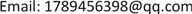1. 引言

f ( x ) = 〈 w , x 〉 + b ,   w ∈ R n , b ∈ R .

min w , b 1 2 ‖ w ‖ 2

s.t.

{ y i − 〈 ω , x i 〉 − b ≤ ε 〈 ω , x i 〉 + b − y i ≤ ε i = 1 , 2 , ⋯ , l

| ξ | ε : = { 0 ,                     如 果 | ξ | ≤ ε | ξ | − ε ,             否 则

min w , b 1 2 ‖ w ‖ 2 + C ∑ i = 1 l ( ξ i + ξ i * )

s.t.

{ y i − 〈 w , ϕ ( x i ) 〉 − b ≤ ε + ξ i 〈 w , ϕ ( x i ) 〉 + b − y i ≤ ε + ξ i * ξ i , ξ i * ≥ 0 .

max W ( α , α * ) = − 1 2 ∑ i , j = 1 l ( α i , α i ∗ ) ( α j − α j ∗ ) K ( x i , x j )     + ∑ i = 1 l ( α i − α i ∗ ) y i − ∑ i = 1 l ( α i + α i ∗ ) ε

∑ i = 1 l ( α i − α i ∗ ) = 0 ,   α i + α i * ≤ C ,   i = 1 , ⋯ , l

α i , α i * ≥ 0 ,   i = 1 , ⋯ , l .

f ( x ) = ∑ i = 1 l ( α i − α i ∗ ) K ( x , x i ) + b .

2. 模型建立

3. 模型实现3.1. 数据选取

3.2. 数据预处理

x i = x i − x mid x max − x min

x i 表示归一化的数据中的第i个数值， x max 为每项归一化数据中的最大值， x min 为每项归一化数据中的最小值， x mid 为区间中间值这里取0为中间值,这里的区间取得是[−2, 2]。

3.3. 参数优化选择

3.4. 利用最佳参数训练SVM模型做拟合预测

4. 实验结果

5. 结论